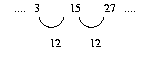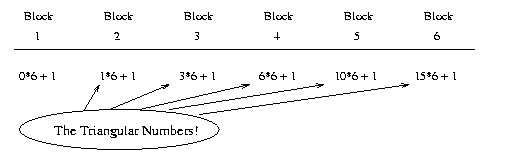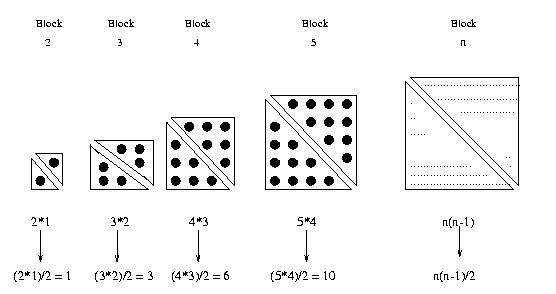# An Investigation Based on Score

##### Age 11 to 14

Published 1999 Revised 2010

Many of you will remember a problem entitled "Score" which recently appeared in the June Six. Class 2YP from Madras College was inspired by this to work out in how many ways the number 1999 could be expressed as the sum of 3 odd numbers, and this is their solution.

We decided to investigate the number of different ways various totals may be obtained by adding small numbers of odd numbers. Systematic working led us to consider the cases: 1 odd number, 2 odd numbers, 3 odd numbers.

### 1 odd number

There was unanimous agreement! Each of the totals 1,3,5,7,... can be obtained in only one way. The pattern is:

1,1,1,1,1,1,1,......

with repeating block .

### 2 odd numbers

Eventually there was unanimous agreement:

 Totals: 2 4 6 8 10 12 14 Ways: 1+1 3+1 5+1 7+1 9+1 11+1 13+1 3+3 5+3 7+3 9+3 11+3 etc... 5+5 7+5 9+5 7+7 1 1 2 2 3 3 4

and a repeating block pattern emerges. In this case the 1st differences are repeated:

 1 1 2 2 3 3 4 ... 0 1 0 1 0 1 ...

with repeating block in the 1st differences of .

### 3 odd numbers

There was some agreement and much confusion. What emerged was the following:

 Totals: 3 5 7 9 11 13 15 Ways: 1+1+1 3+1+1 5+1+1 7+1+1 9+1+1 11+1+1 13+1+1 3+3+1 5+3+1 7+3+1 9+3+1 11+3+1 3+3+3 5+5+1 7+5+1 9+5+1 5+3+3 7+3+3 9+3+3 5+5+3 7+7+1 7+5+3 5+5+5 1 1 2 3 4 5 7

There was initial excitement when 1,1,2,3,... emerged and Fibonacci's name was bandied about.

By the time 1,1,2,3,4,5,... was reached the initial 1 was being viewed as a "rogue" value and most of the class were in agreement with 6,7,8,... being the continuation. There was great consternation when 7 emerged and not 6.

Eventually this pattern emerged:

 1 1 2 3 4 5 7 8 10 12 14 16 19 21 24 27 30 0 1 1 1 1 2 1 2 2 2 2 3 2 3 3 3 1 0 0 0 1 -1 1 0 0 0 1 -1 1 0 0

and so the conjecture emerged that there was a repeating block in the 2nd differences of [1,0,0,0,1,-1].

" In how many ways can 1999 be written as the sum of three odd numbers? "

Agreement was reached that continuing the pattern to reach 1999 was not feasible!

A fresh look at the block structure:...

 3 5 7 9 11 13 15 17 19 21 23 25 27 29 ... ---------------------- -------------------------- --------- 1 1 2 3 4 5 7 8 10 12 14 16 19 21 ... 0 1 1 1 1 2 1 2 2 2 2 3 2 3 ... 1 0 0 0 1 -1 1 0 0 0 1 -1 1 0 ... ------------------------- -------------------------- --------- B L O C K 1 B L O C K 2

raised a new question: In which block is 1999?

The first "total" in each block givesSo comparing with the multiples of 12 ... 12, 24, 36, ... gave us the formula 12n-9 for the first "total" on block n.

Now 12n-9=1999 => 12n=2008 => n=167.333...

So 1999 is in the 167th block. 12 * 167 - 9 = 1995 gives :

 1995 1997 1999 ... - - - - - - - - ? 166 167 167 ... 1 0 0 ... - - - - - - - -
So what number could be in the first row of block 167? Here are the numbers at the start of the first row:

 Block 1 Block 2 Block 3 Block 4 ... Block 167 - - - - - - - - - 1 7 19 37 ... ? 6 12 18 ... 6 6 ...
At this point we were all set to do a lengthy extension of the pattern when Sophie Palmer spotted the following pattern:We tackled this geometrically:So the formula for the first number in the nth block is:

$6n(n-1)/2 + 1$
$= 3n(n-1) + 1$
$= 3n^2 - 3n + 1$

In particular when $n=167$ this gives $3*167^2 - 3*167 + 1 = 83167$

We now have:
 1995 1997 1999 - - - - - - - 83167 83333 83500 ... 166 167 167 ... 1 0 0 ... - - - - - - - -
So class 2YP (with a little help from Mr Nisbet!) discovered that 1999 can be written as the sum of three odd numbers in exactly 83500 different ways. Their homework for Monday night is to write them all out!
Class 2YP has clearly done an impressive job. Well done to them and to anyone else who tackled this problem and got the right answer.